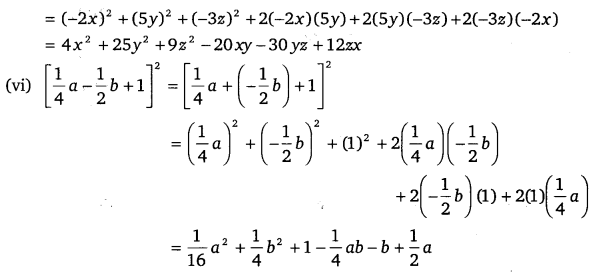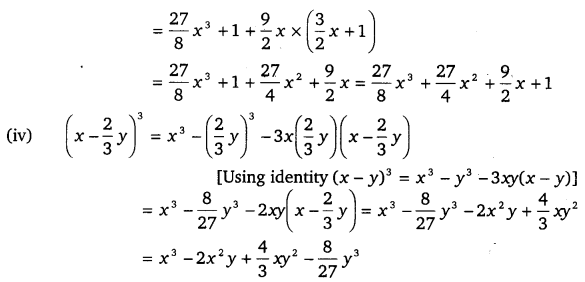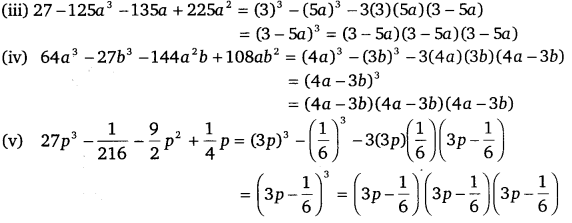# CLASS 9 MATH NCERT SOLUTION FOR CHAPTER – 2 POLYNOMIALS EX – 2.5

## Polynomials

Question 1.
Use suitable identities to find the following products:
(i) (x+4)(x+10)
(ii) (x+8)(x-10)
(iii) (3x+4)(3x+2x)
(iv) (${ y }^{ 2 }+\cfrac { 3 }{ 2 }$)(${ y }^{ 2 }-\cfrac { 3 }{ 2 }$)
(v) (3-2x)(3+2x)

Solution:

Question 2.
Evaluate the following products without multiplying directly:
(i) 103 x 107
(ii) 95 x 96
(iii) 104 x 96

Solution:
(i) 103 x 107 = (100 + 3) (100 + 7)
= (100)2 + (3 + 7) (100) + 3 x 7
= 100 x 100 + (10)(100) + 21
= 10000 +1000 + 21 = 11021

(ii) 95 x 96 = (100 – 5) (100 – 4)
= (100)2 + (-5 – 4)(100) + (-5)(-4) – 100 x 100 + (-9)(100) + 20
= 10000 – 900 + 20 = 9120

(iii) 104 x 96 = (100 + 4)(100 – 4)
= (100)2 – (4)2
= 10000 -16
= 9984

Question 3.
Factorise the following using appropriate identities :
(i) 9x2 + 6xy + y2
(ii) 4y2 – 4y +1
(iii) x2$\cfrac { { y }^{ 2 } }{ 100 }$

Solution:

Question 4.
Expand each of the following, using suitable identities :
(i) (x + 2y + 4z)2
(ii) (2x -y +z)2
(iii) (-2x + 3y + 2z)2
(iv) (3a – 7b -c)2
(v) (-2x + 5y – 3z)2
(vi)$(\cfrac { 1 }{ 4 } a-\cfrac { 1 }{ 2 } b+1)$2

Solution:Question 5.
Factorise:
(i) 4x2 + 9y2 + I622 + 12xy – 24yz -16xz
(ii) 2x2+ y2 + 822 – 2$\sqrt { 2 }$xy + 4$\sqrt { 2 }$yz – 8×2

Solution:
(i) 4x2 +9y2 +16z2 +12xy-24yz-16xz
= (2x)2 + (3y)2 + (-4z)2 + 2(2x)(3y) + 2(3y)(-4z) + 2(-4z)(2x)
= (2x +3y – 4z)2

(ii) 2x2 + y2 + 8z2 – 2$\sqrt { 2 }$xy + 4$\sqrt { 2 }$yz-8xz
= (-a$\sqrt { 2 }$x)2 + (y)2 + (2$\sqrt { 2 }$z)2 + (2 –$\sqrt { 2 }$x) (y) + 2(y) (2$\sqrt { 2 }$z) + 2(2$\sqrt { 2 }$z)(-$\sqrt { 2 }$x)
= (-$\sqrt { 2 }$x + y + 2$\sqrt { 2 }$z)2

Question 6.
Write the following cubes in expanded form :
(i) (2x+1)3(ii) (2a-3b)3
(iii)$(\cfrac { 3 }{ 2 } x+1)$3
(iv)$(x-\cfrac { 2 }{ 3 } y)$3

Solution:Question 7.
Evaluate the following using suitable identities :
(i) (99)3
(ii) (102)3
(iii) (998)3

Solution:

Question 8.
Factorise each of the following:
(i) 8a3 + b3 + 12a26 + 6ab2
(ii) 8a3 – b3 -12a26 + 6a62
(iii) 27 – 125a3 – 135 a + 225 a64a3
(iv)$27{ p }^{ 3 }-\cfrac { 1 }{ 216 } -\cfrac { 9 }{ 2 } { p }^{ 2 }+\cfrac { 1 }{ 4 } p$

Solution:Question 9.
Verify:
(1) x3 + y3 = Or + y)(x2-xy + y2)
(ii) x3-y3 = (x-y)(x2 + xy + y2)

Solution:
(i) We know that,
(x + y)3 = x3 + y3 + 3xy(x + y)
=> x3 + y3 = (x + y)3 -3xy(x + y)
= (x + y)[(x + y)2 -3xy]
= (x + y) [x2 + y2 + 2xy – 3x] = (x + y)[x2 + y2 – xy]
= RHS  Hence proved.

(ii) We know that, (x – y)3 = x3 – y3 -3xy(x – y)
=>x3 – y3 = (x – y)3 + 3xy(x – y)
= (x-y)[(x – y)2 +3xy]
= (x -y)[x2 + y2 -2xy + 3xy]
= (x — y)[x2 + y2 + xy]
= RHS  Hence proved.

Question 10.
Factorise each of the following:
(i) 27y3 + 125z3
(ii) 64m3 -343n[Hint: See question 9]

Solution:
(i) 27 y3 +125z3 = (3y)3 + (5z)3
= (3y + 5z)[(3y)2 – (3y)(5z) + (5z)2]
= (3y + 5z) (9 y2 – 15yz + 25z2)

(ii) 64m3 -343n3 = (4m)3 -(7n)3
= (4m-7n)[(4m)2 + (4m)(7n) + (7n)2]
= (4m – 7n)[16m2 + 28mn + 49n2]

Question 11.
Factorise :
27x3 + y3 + z3 – 9xyz

Solution:
27x3 +y3 +z3 -9xyz = (3x)3 + y3 +z3 -3(3x)(y)(z)
= (3x + y + z)[(3x)2 + y2 + z2 – (3x)y – yz -z(3x)]
= (3x + y + z)(9x2 + y2 + z2 -3xy – yz -3zx)

Question 12.
Verify that
x3 + y3 +z3 -3xyz =$\cfrac { 1 }{ 2 }$ (x + y + z)[(x -y)2 +(y-z)2 +(z-x)2]

Solution:
We have, x3 + y3 + z3 – 3xyz
= (x + y + z) [x2 + y2 + z2 – xy – yz – zx]$\frac { 1 }{ 2 }$(x + y+ z)[2x2 +2y2 +2z2 -2xy-2yz -2zx]$\frac { 1 }{ 2 }$(x + y + z)[x2 + x2 + y2 + y2 + z2 + z2 -2xy-2yz-2zx]$\frac { 1 }{ 2 }$(x + y + z)[x2 + y2 – 2xy + y2 + z2 -2yz + z2 + x2 – 2zx]$\frac { 1 }{ 2 }$(x + y + z)[(x-y)2 + (y-z)2 +(z-x)2]

Question 13.
If x + y + z = 0, show that x3 + y3 + z3 = 3xyz.

Solution:
We know that,
x3 +y3 + z3 – 3xyz = (x + y + z)(x2 + y2 + z2 – xy – yz-zx)
= 0(x2 + y2 + z2 – xy- yz-zx) (∵ x + y + z = 0 given)
= 0
=> x3 + y3 + z3 = 3xyz        Hence proved.

Question 14.
Without actually calculating the cubes, find the value of each of the following:
(i) (-12)3 + (7)3 + (5)3
(ii) (28)3 + (-15)3 + (-13)3.

Solution:
(i) We know that, x3 + y3 + z3 – 3xyz
= (x + y + z)(x2 + y2 + z2 – xy – yz – zx)
Also, we know that, if
x + y + z = 0
Then, x3 + y3 +z3 = 3 xyz
Given expression is (-12)3 + (7)3 + (5)3.
Here,            -12 + 7 + 5=0
∴ (-12)3 + (7)3 + (5)3 = 3 x (-12) x 7 x 5 = -1260

(ii) Given expression is (28)3 + (-15)3 + (-13)3
Here, 28 + (-15) + (-13) = 28 -15-13 = 0
∴  (28)3 + (-15)3 + (-13)3 = 3 x (28) x (-15) x (-13) = 16380

Question 15.
Give possible expressions for the length and the breadth of each of the following rectangles, in which their areas are given :
(i) Area = 25a2 – 35a + 12
(ii) Area = 35y2 + 13y – 12.

Solution:
(i) We have,
Area of rectangle = 25a2 – 35a +12 [by splitting the middle term]
= 25a2 -20a-15a+12
= 5a(5a – 4) – 3(5a – 4)
= (5a-4)(5a-3)
Hence, possible expression for length = (5a – 3) and possible expression for breadth = (5a – 4)

(ii) We have,
Area of rectangle = 35y+ 13y -12
= 35y2 + (28 – 15)y -12
= 35y2 + 28y-15y-12
= (35y2 +28y)-(15y + 12)
= 7y(5y + 4) – 3(5y + 4)
= (7y – 3) (5y + 4)

Question 16.
What are the possible expressions for the dimensions of the cuboids whose volumes are given below?
(i) Volume = 3x2 – 12x
(ii) Volume = 12ky2 + 8ky – 20k.

Solution:
(i) We have,
Volume of cuboid = 3x2 -12x = 3x(x – 4)
So, the possible expressions for the dimensions of the cuboids are 3, x and x – 4.
[∵ volume of cuboid = length x breadth x height]

(ii) We have,
Volume of cuboid = 12ky2 + 8ky – 20k = 12ky2 + (20 -12)ky – 20k [by splitting the middle term]
= 12ky2 + 20 ky – 12ky – 20 k = 4ky(3y + 5) – 4k(3y + 5) = (3y + 5)(4ky – 4k)
= (3y + 5)4k(y -1) = 4k(3y + 5)(y -1)
So, the possible expressions for the dimensions of the cuboid are 4 k, 3y + 5 and y -1.

### Take Your IIT JEE Coaching to Next Level with Examtube

• Mentoring & Teaching by IITians
• Regular Testing & Analysis
• Preparation for Various Engineering Entrance Exams
• Support for School/Board Exams
• 24/7 Doubts Clarification